﻿ ANSDIMAT+ > Aquifer tests analysis > Matching parameters (solving direct problem) > Parameter calculation

# Parameter calculation

The "Parameter calculation" dialog window is a simple calculator for aquifer parameters. It supports the calculation of parameters including the leakage factor, hydraulic diffusivity, transmissivity, retardation coefficient (for pumping near river) and piezometer shape factor. This last parameter is used not only for calculations but also for defining the piezometer position. This constitute a sensitive parameter for some solutions taking into account storage capacity of the piezometer (see Moench’s solutions for confined and unconfined aquifer).

Leakage factor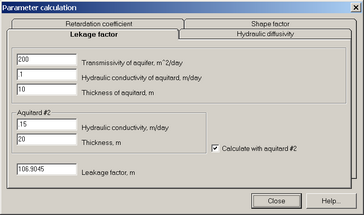Leakage factor calculation dialog.

Equations for leakage factor calculation: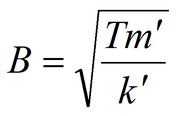one aquitard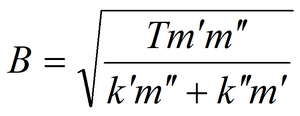two aquitards – double prime relates to the parameters of the second aquitard

Hydraulic diffusivity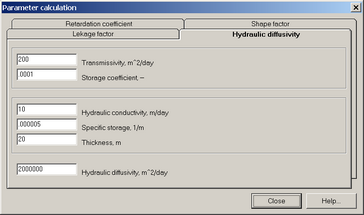Hydraulic diffusivity calculation dialog.

Equation for calculating hydraulic diffusivity: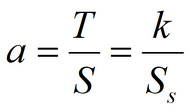storage coefficient:In this dialog parameters are automatically inter-calculated (i.e. when Hydraulic Cobductivity and thickess are input, Transmissivity is re-calculated).

Retardation coefficientRetardation coefficient calculation dialog.

Equation for retardation coefficient calculation (in Shestakov’s solution):Equation for retardation coefficient calculation (in Hantush’s solution):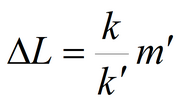Where T - aquifer transmissivity, m2/day; k - aquifer hydraulic conductivity, m/day; k' - hydraulic conductivity of the river bed, m/day; m' - river bed thickness, m; b - river width, m.

Shape factorShape factor calculation dialog.

This tab allows selecting the piezometer configuration in solutions accounting for piezometer storage capacity (see Moench’s solutions for confined and unconfined aquifer with account for both piezometer and pumping well storage capacity). Furthermore, the user can estimate the shape factor value at a specific well radius, screen length, aquifer thickness (or initial water saturated thickness of unconfined aquifer), anisotropy and piezometer configuration.

"Modified" option calculates the shape factor used in Moench’s solution: shape factor divided by 2π